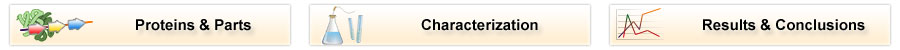# Team:TU Delft/Project/rbs-characterization/characterization

(Difference between revisions)
 Revision as of 14:14, 22 October 2010 (view source)← Older edit Revision as of 14:39, 22 October 2010 (view source)Newer edit → Line 73: Line 73: :Jason R. Kelly, Adam J. Rubin, Joseph H. Davis, ''et al'' (March 2009). "[http://dx.doi.org/10.1186/1754-1611-3-4 Measuring the activity of BioBrick promoters using an in vivo reference standard]". ''Journal of Biological Engineering'' '''3''': 4 :Jason R. Kelly, Adam J. Rubin, Joseph H. Davis, ''et al'' (March 2009). "[http://dx.doi.org/10.1186/1754-1611-3-4 Measuring the activity of BioBrick promoters using an in vivo reference standard]". ''Journal of Biological Engineering'' '''3''': 4 - ===Continue Reading about the RBS Characterization===

## Characterization### RBS strength

The RBS strength defines how much of a protein is produced compared to a reference RBS sequence. However, RBS characterization measurements only include current protein level (GFP measurements) and current biomass concentration (Absorption measurements). During measurements, the protein concentration is also influenced by other factors:

• The rapid growth of the bacteria will dilute the protein considerably

### Known methods

The conventional method for characterization of RBS or promotor sequences is to assume that the derivative of GFP concentration is the production rate, as shown in the formula from Kelly et al (shown below).This method only works if cells are subcultured a few times in the exponential phase. In that case, steady state GFP concentration can be assumed and dilution can be ignored. In our experiment however, this was not the case. Specific GFP is actually decreasing because of dilution, so it needs to be modeled in order to make any use of the data.

### Growth curve fitting

From the biomass, or optical density (OD), graph below, it can be seen that the fastest growth occurs from 1 hour until about 3 hours into the experiment. Within this timespan, it is assumed that growth is exponential, and growth rate can be calculated. The graph below shows 72 growth curves. 12 seperate wells were used for every RBS sequence.

The exponential part of this graph is curve-fitted to an exponential growth model:

Which results in a growth rate ('mu') that can be used to add dilution to further calculation.

### Biomass growth will dilute the GFP

At the start of our measurements, the GFP protein has reached a steady state high concentration. The specific GFP will decrease during growth because of dilution, and this needs to be accounted for when calculating the RBS strength.

## Protein production model

As said, the protein production model needs to take dilution into account. The GFP protein that is used is very stable (half life 41 hours), so the degradation term is negligible compared to dilution.

In above formula:

• Y is the GFP concentration
• Beta is the production rate (The constant that we are interested in)
• Alpha is the dilution+degradation rate

Converting this equation to an explicit form results in:

The 2 unknown parameters (beta and Y1) where curve-fitted against the GFP/OD data, using the matlab lsqcurvefit command. The beta constant for each of the 72 experiments were then to calculate RBS strengths, listed in the results page.

### Data normalization method

To go from original measurements to RBS strenghts, we ran the following steps using a matlab script:

Our plate reader data consists of 12 columns of

• 5 strains with Anderson RBS'es to be characterized
• B0032 strain to compare to
• I13401 strain as control (GFP without promotor and RBS)
• LB+Amp as blank

To prepare the data for further calculation, some things have to be done:

• Subtract the blank GFP and OD values from the data with E coli in it. This takes care of the platereader error independent of biomass
• Biomass without GFP also produces some nonzero measurements (See right). From the control strain measurements we can calculate how much 'fake' GFP is reported due to biomass.
• For each well, we calculate the real GFP that is not a result of biomass:
```real_gfp = gfp - fake_gfp_per_od * od
```
• These values are curve fitted into our protein production model, resulting in a (exponential phase) protein production rate for each well.